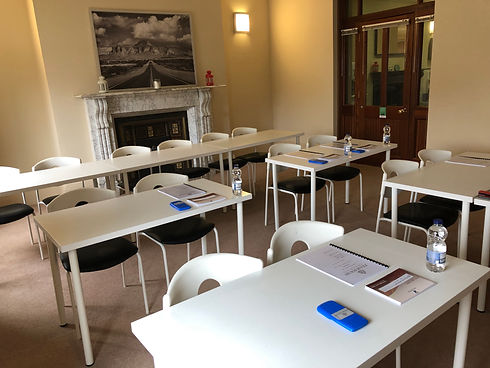top of page

## 3rd Year Higher Level Maths

• Trigonometry

• Geometry

• Coordinate Geometry

• Arithmetic

• Area & Volume

• Functions

• Equations

## 6th Year Ordinary Level Maths

Paper 1

• Algebra

• Indices

• Arithmetic (Financial Maths)

• Complex Numbers

• Functions

• Differentiation

• Relevant exam questions associated with all of the above.

• All other "must know" questions and formulae will also be covered.

Paper 2

• Geometry constructions 16 - 21.

• Statistics

• Probability

• Geometry & Enlargements

• Coordinate Geometry of the Line & Circle

• Applied Measure (Area & Volum)

• Relevant exam questions associated with all of the above.

• All other "must know" questions and formulae will also be covered

## 6th Year Higher Level maths

Paper 1

• Proof by induction (Series/Divisibility/Inequalities/De Moivre's Theorem).

• Using De Moivre's Theorem to Prove Trigonometric Identities.

• Proof by contradiction (Root 2/Root 3).

• Construction of Root 2 and Root 3.

• Derivation of the Sum to Infinity Formula.

• Derivation of the Amortisation Formula.

• Relevant exam questions associated with all of the above.

• All other "must know" questions and formulae will also be covered.

Paper 2

• Geometry constructions 16 - 22.

• Formal proofs of theorems 11, 12 & 13.

• Trigonometry derivations 1 - 7 & 9.

• Relevant exam questions associated with all of the above.

• All other "must know" questions and formulae will also be covered

## 6th Year Higher Level Chemistry

• Question 1 (The "most likely" Titration questions)

• Question 2 (The "most likely" Organic Experiment Questions)

• Question 3 (The "most likely" Miscellaneous Experiment Questions)

• Fuels & Heats of Reaction

• Organic Chemistry

• Equilibrium

• pH and Water

#### Booking Form

Please fill out the form below to register your interest for any of the above courses. We will then contact you to confirm your place. Once dates, times and topics have been confirmed we will contact you with this update. You can opt-out of the course at this point if dates/times do not suit.###### Cost

1 Intensive: €80

2 Intensives: €150

###### What to Bring

Pencil Case.

Calculator.

Formulae & Tables Book

bottom of page